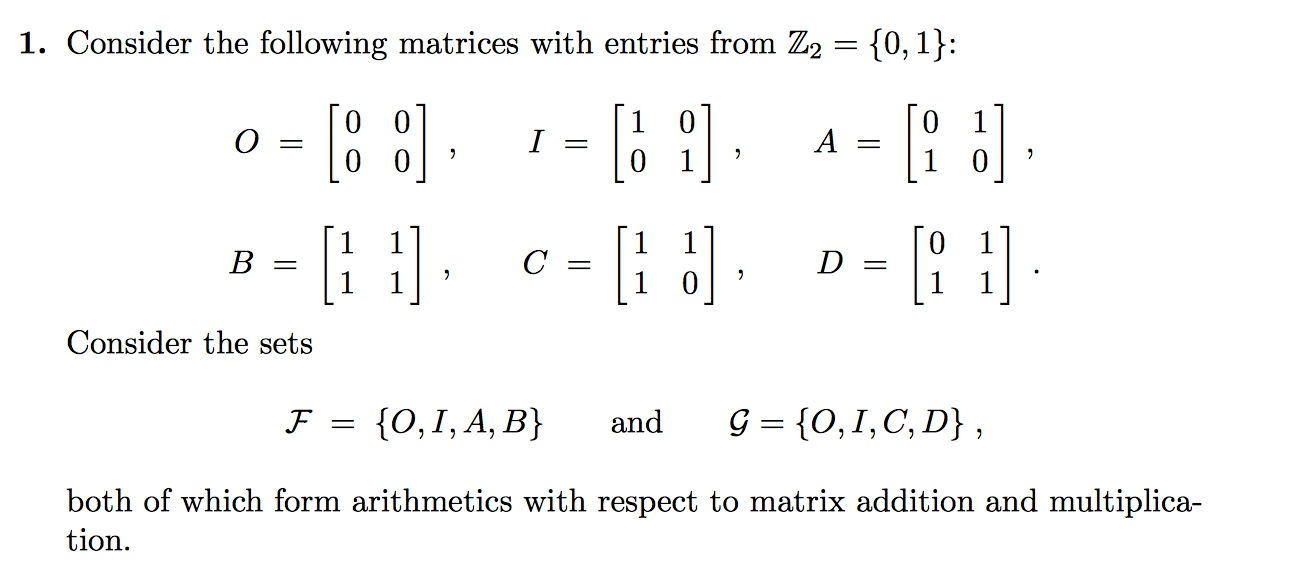# 1. Consider the following matrices with entries from Z2 - 10, 1)D=Consider the setsboth of which form arithmetics with respect to matrix addition and multiplica-tion

Question
63 views

Explain why G forms a field with respect to addition and multiplication. (You may quote relevant properties of matrix addition and multiplication without proof.)
Why the arithmetic F does not form a field?help_outlineImage Transcriptionclose1. Consider the following matrices with entries from Z2 - 10, 1) D= Consider the sets both of which form arithmetics with respect to matrix addition and multiplica- tion fullscreen
check_circle

Step 1

The two sets are

Step 2

See to the definition of field, a set F is a field that has atleast two elements operated with addition or multiplication such that for each pair of elements a, b belongs to F, there exist unique elements a + b belongs to F and ab belongs to F. For a set F to be a field, each element in F has an additive inverse and a multiplicative inverse in F.

Additive inverse exists for each element in F and G but it is important to see the result for multiplicative inverse.

For C and D in G, CD belongs in G. For multiplicative inverse of D, observe that

Step 3

Here, multiplicative inverse of D is C and simultaneously, multiplicative inverse of C is D.

Therefore, G is a Field as both additive and...

### Want to see the full answer?

See Solution

#### Want to see this answer and more?

Solutions are written by subject experts who are available 24/7. Questions are typically answered within 1 hour.*

See Solution
*Response times may vary by subject and question.
Tagged in